go back to main

5.4. Real data with  feature selection

 Real data of object images (COIL-100)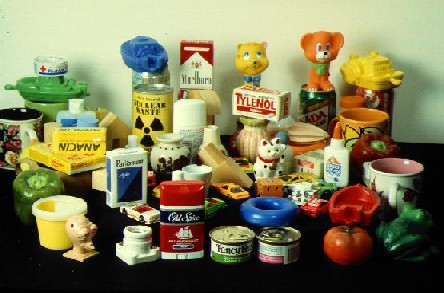Real data of face images (ORL faces)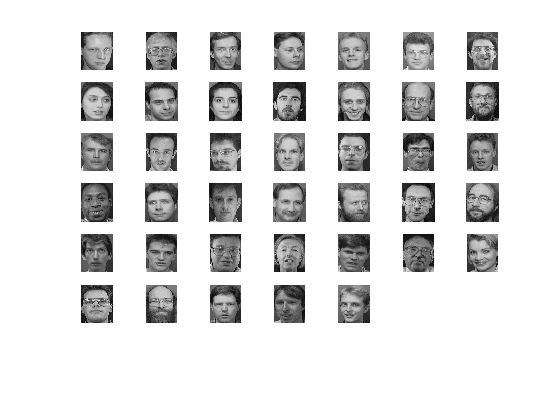With the feature selection  parameter set, the matlab code  is limited to 8 components due to computational time costs (n_vectors=7 or less).

EXPERIMENT 17

20 first objects from COIL-100 and FASTICA

objects=20;
x=data_coil100(objects);
%%%%%%%%%%%%%%%%%%%%%%%%%%%%%%%%%%%%%%%%%%%%%%%%%%
nc=objects; %number of classes
np=10; %number of samples per class
n_vectors=6; %number of vectors used to reduce the data
nr=6; %number of repetitions of the classification process (DIFFERENT EXPERIMENTS)
per_test=0.5; %percentage of test samples from x (0-1)

>> example(3,1,1)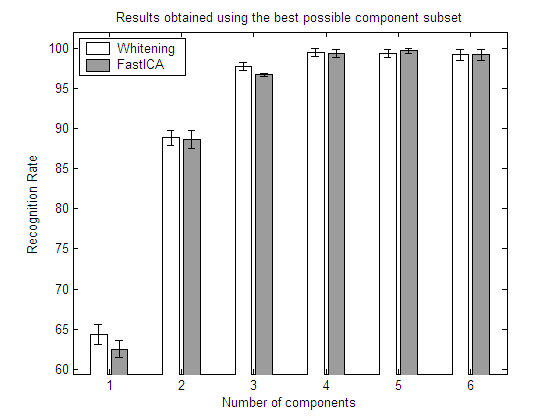EXPERIMENT 18

20 first objects from COIL-100   and INFOMAX

objects=20;
x=data_coil100(objects);
%%%%%%%%%%%%%%%%%%%%%%%%%%%%%%%%%%%%%%%%%%%%%%%%%%
nc=objects; %number of classes
np=10; %number of samples per class
n_vectors=6; %number of vectors used to reduce the data
nr=6; %number of repetitions of the classification process (DIFFERENT EXPERIMENTS)
per_test=0.5; %percentage of test samples from x (0-1)

>> example(3,2,1)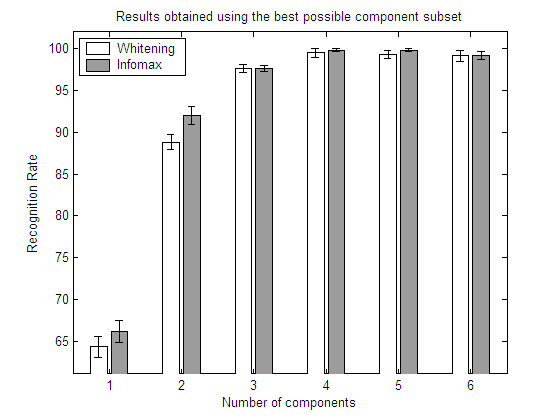EXPERIMENT 19

40 people from ORL and FASTICA

%%%%%%%%%%%%%%%%%%%%%%%%%%%%%%%%%%%%%%%%%%%%%%%%%%%%
% Images from ORL FACE DATABASE %%%%http://people.cs.uchicago.edu/~dinoj/vis/ORL.zip
objects=40;
np=10; %number of samples per class
x=data_orl_faces(objects,np,see);
%%%%%%%%%%%%%%%%%%%%%%%%%%%%%%%%%%%%%%%%%%%%%%%%%%
nc=objects; %number of classes
n_vectors=5; %number of vectors used to reduce the data
nr=20; %number of repetitions of the classification process

per_test=0.5; %percentage of test samples from x (0-1)

>> example(4,1,1)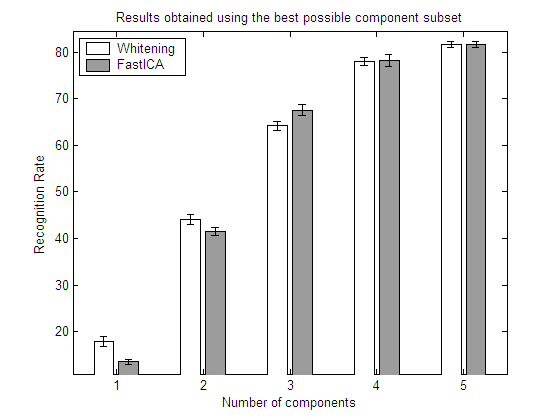EXPERIMENT 20

40 people from ORL and INFOMAX

>> example(4,2,1)

%%%%%%%%%%%%%%%%%%%%%%%%%%%%%%%%%%%%%%%%%%%%%%%%%%%%
% Images from ORL FACE DATABASE %%%%http://people.cs.uchicago.edu/~dinoj/vis/ORL.zip
objects=40;
np=10; %number of samples per class
x=data_orl_faces(objects,np,see);
%%%%%%%%%%%%%%%%%%%%%%%%%%%%%%%%%%%%%%%%%%%%%%%%%%
nc=objects; %number of classes
n_vectors=5; %number of vectors used to reduce the data
nr=20; %number of repetitions of the classification process

per_test=0.5; %percentage of test samples from x (0-1)CONCLUSIONS (Experiments 17-20)

In the above graphs,  for each number of components returned by the algorithm, the best possible set is selected by means of an exhaustive feature selection process. In this way, the results show the recognition rates that could be obtained with each algorithm if a perfect selection of components is carried out.

The results show clearly that a feature selection process may have a strong influence in the recognition rates obtained by each algorithm. FastICA and whitened PCA no longer perform equally; and the same applies to Infomax. Eventhough the results of the three algorithms differ to a great extent, there is no clear winner. As all the algorithms are unsupervised, the results are highly dependent on the class distributions. This fact was previously shown in section 5.3.  with the artificial datasets.

go back to main